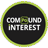Compound InterestQuantitative Aptitude Questions and Answers section on “Compound Interest” with solution and explanation for competitive examinations such as CAT, MBA, SSC, Bank PO, Bank Clerical and other examinations.

In how many years will a sum of money 800 Rs. at 10% per annum compound interest, compounded semi annually becomes 926.10 Rs.?

In how many years will a sum of money 800 Rs. at 10% per annum compound interest, compounded semi annually becomes 926.10 Rs.? [A]$latex 1\frac{2}{3}\ years&s=1$ [B]$latex 1\frac{1}{2}\ years&s=1$ [C]$latex 2\frac{1}{3}\ years&s=1$ [D]$latex 2\frac{1}{2}\ years&s=1$ Show Answer $latex \mathbf{1\frac{1}{2}\ years}&s=1$ Rate = 10% per annum = 5% half yearly \$latex \because A = P(1 + ..